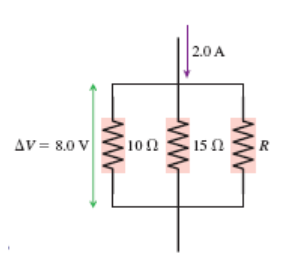# Problem: What is the value of resistor R in the figure below?A) 4.0 ΩB) 12 Ω   C) 36 ΩD) 72 ΩE) 96 Ω

###### FREE Expert Solution

Ohm's law:

$\overline{){\mathbf{V}}{\mathbf{=}}{\mathbf{i}}{\mathbf{R}}}$

Equivalent resistance for parallel resistors:

$\overline{)\frac{\mathbf{1}}{{\mathbf{R}}_{\mathbf{e}\mathbf{q}}}{\mathbf{=}}\frac{\mathbf{1}}{{\mathbf{R}}_{\mathbf{1}}}{\mathbf{+}}\frac{\mathbf{1}}{{\mathbf{R}}_{\mathbf{2}}}{\mathbf{+}}\frac{\mathbf{1}}{{\mathbf{R}}_{\mathbf{3}}}}$

Using ohm's law:

Req = V/i = 8.0/2.0 = 4 Ω

81% (27 ratings)###### Problem Details

What is the value of resistor R in the figure below?

A) 4.0 Ω

B) 12 Ω

C) 36 Ω

D) 72 Ω

E) 96 ΩFrequently Asked Questions

What scientific concept do you need to know in order to solve this problem?

Our tutors have indicated that to solve this problem you will need to apply the Combining Resistors in Series & Parallel concept. You can view video lessons to learn Combining Resistors in Series & Parallel. Or if you need more Combining Resistors in Series & Parallel practice, you can also practice Combining Resistors in Series & Parallel practice problems.

What professor is this problem relevant for?

Based on our data, we think this problem is relevant for Professor Haselwandter's class at USC.